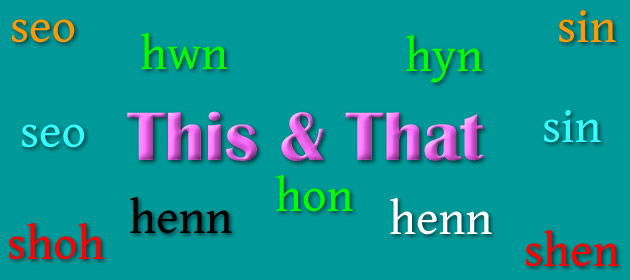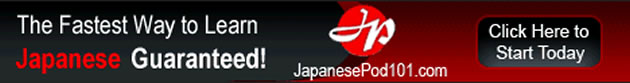# This & That

Words for this and that in Celtic languages.Proto-Celtic *so [so] = this so = this so, sa, se, sea, seo, siu = this seo [ʃɔ] = this, here anseo = here, in this place go dtí seo = up to now faoi seo = by now roimhe seo = before this, formerly seo dhuit = here, take it seo linn = here we go seo leat = come on seo d’am (agat)! = now is your chance! seo [ʃɔ] = this, these seo, seo … = (well) now then, … an-seo = here an déidh seo = after this, afterwards, hereupon, later on chuige seo = hitherto, until now feadh an-seo = around here, hereabouts gu seo = up until now roimhe seo = before, by now shoh = this, this here ad shoh = those, those here ayns shoh = here myr shoh = in this manner, thus roish shoh = previously (yn chiaghtin) shoh cheet = coming / next (week)

Etymology: from the Proto-Indo-European *só (this, that), which is also the root of the English words this, that, the, then, than and there [source].

Proto-Celtic *sindos = this *sondo- = that sinde, sindas = that sin [sʲinʲ] = that in [inʲ] = the sin [ʃɪnʲ / ʃɨ̞nʲ] = that an [ənˠ / ə.n̠ʲ / ə] = the ó shin = ago, back, since then, from then mar sin = like that, thus mar sin de = in that case, therefore agus mar sin de = and so on go dtí sin = up to that point, until then iar sin = after that, thereupon roimhe sin = before that faoi sin = by then sin [ʃin] = that, those, there an, am, a’ = the an-sin = there, therein, thither, then sin thu! = well done, bravo!, well done mar sin dheth = consequently …, so, therefore uime sin = therefore, thereupon, then shen = that yn = the ad shen = those ayns shen = there myr shen = in that manner, so, therefore, thus roish shen = before then, prior to that hinn = this, that ir = the hvnn, hunn, hun, hon, hwnn = this, that y, yr, ‘r = the hyn [hɨ̞n /hɪn] = this, these, this time,this place, these, they; such (a), so much, so many hynny = that, that time, that place, that number/amount, those hyn a hyn = so much, so many, a certain quantity, such and such ar hyn o bryd = now, at this (point in) time, at the present moment hwn [hʊn] = this (one), he (him, she (her), it; that (with masculine nouns) hwnnw, hwnna = that hwn a hwn = such a one, such as such a person, so-and-so hwn/hon/hyn a’r llall = this and that; this, that and the other hon [hɔn] = that (with feminine nouns) honno, honna = that y, yr, ‘r = the hen = this henna = the one there, that one, that homma = this female here, this one, this hon = this female, this an = the henn, hedn = that henna, honna = that (one) dres henna = moreover, besides wosa henna = after that, later, thereafter an = the henn, hen = that, after that an, ar, al = the an, ar, al = the

Etymology: from the Proto-Indo-European *sḗm (one) or *só (this, that) [source].

Old Irish (Goídelc) siút, sút = that, yon út = yon, yonder siúd [ʃuːd̪ˠ] = that, yon ansiúd = yonder, there beyond úd = yon, yonder, that … over there siud [ʃid] = that (over there), yon (those) an-siud = there, yonder shid = yonder, that ad shid = those ayns shid hoal = over yonder

Etymology: uncertain

Words marked with a * are reconstructions.## One thought on “This & That”

1.Robert says:

Simon, a (really) long time ago, someone tried to advise me not to pursue wealth. The advise was, “What good is a bunch of money, anyway? All it will buy you is a bunch of stuff and things.”

After many years, the expression “stuff and things” has stuck with me, much more so than any mere, mundane thing like “this and that”. I mean, look at you: You wrote a bunch of stuff and things about Celtic languages.

Not just about money, it’s a good, all-purpose expression well worth adopting :-))

Cheers,

Robert